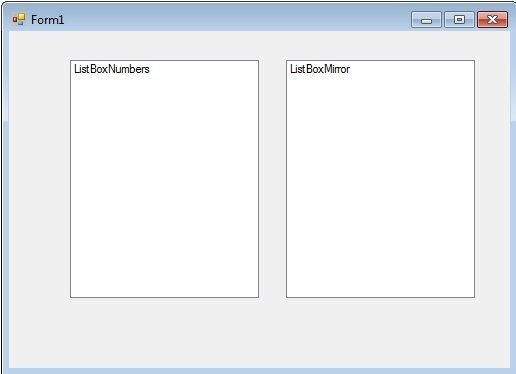# Visual Basic For Loop

This third tutorial on loops, following the Do While and the Do Until loop, covers the For Loop.  There are two For Loops in Visual Basic, the For Next Loop, which you’ll see in virtually every programming language including .NET, Visual Basic 6.0, VB Script and VBA, and the For Each loop which was introduced to VB.NET.

The For Loop is my favourite of all the loops and one which I use far more than the two Do Loops.   The For Loop is designed for looping through some sort of sequence.  That sequence may be 1 through to 50, or items in a collection.  With the For Loop you define the sequence and iterate through it.

The syntax for the For Next loop is as follows

```For number = lower value To higher value

Code

Next```

In practise this looks as follows

```For i As Integer = 1 to 10
Code
Loop```

Most loops are executed over some kind of counter.   If you do want to loop through a counter then I would always go for a For Loop as opposed to one of the two Do loops.  The For loop defines everything you need to know in one line.  You know exactly where you are with the For loop the second you read it.   Everything is there to digest without having to look in the body of the loop.  It’s the most honest loop, is straight to the point and that appeals to me.

Look at this same code with the Do Loop.

```Dim i As Integer

Do While i < 11
Code
i = i + 1
Loop```

Or

```Dim i As Integer

Do Until i = 11
Code
i = i + 1
Loop```

In both cases, you have to look at the first line and the body of code to see how the loop works.  You have the condition in the first line fof the loop and the counter (in this case i = 1 + 1) in the body of the loop.  It’s that much more difficult to read.  The code in both examples works, don’t get me wrong, and working code should be your first priority.  But working code that is easy to read and, hence, easy to maintain, is what you should be aiming for as a craftsman.

You can increment backwards using the For Loop using the following syntax

```For i As Integer = 10 to 1 Step -1
Code
Loop```

Or you can increment by more than 1 if you like using the same instruction

```For i As Integer = 1 to 10 Step 2
Code
Loop```

Create a new Project called “For Loop”.   Add two Listboxes side by side, one called ListBoxNumbers and the other called ListBoxMirror and style them so the Form looks as follows```Dim i As Integer

Do While i < 51
i = i + 1
Loop
```

Now I want you to convert this exact code into a For Next Loop.  No cheating.  Do not look at the answer below.

```For i As Integer = 1 To 50
Next
```

If you didn’t get it, don’t worry, just take a look at the solution.  Hopefully you can immediately see the benefits of this loop.  Instead of 5 lines of code, we have 3.  And to understand the loop, you just read the first line.  You don’t need to look at the body of the loop at all.  It’s a neater and more efficient way or working.

```
Dim listItem As Integer

For i As Integer = 1 To 50
Next

For Each x As Integer In ListBoxNumbers.Items
Next

```

Run this code and you’ll see the two listboxes have exactly the same set of numbers in there.

So what exactly is happening here?

We obviously have two loops here.  The first loop is adding the numbers 1 to 50 to ListBox Numbers.

The second one is a lot more interesting.  The For Each Loop, which is the second of the For Loops, allows you to neatly Loop through every item in a collection.  As ListBoxNumbers is a list if Integers, we are reading each item in sequence, and adding each item to ListBoxMirror.

Change the code in the first loop to this

```For i As Integer = 50 To 1 Step - 1